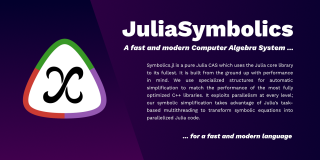# Computer algebra system

Topic | v1 | created by jjones |
Description

A computer algebra system (CAS) or symbolic algebra system (SAS) is any mathematical software with the ability to manipulate mathematical expressions in a way similar to the traditional manual computations of mathematicians and scientists. The development of the computer algebra systems in the second half of the 20th century is part of the discipline of "computer algebra" or "symbolic computation", which has spurred work in algorithms over mathematical objects such as polynomials. Computer algebra systems may be divided into two classes: specialized and general-purpose. The specialized ones are devoted to a specific part of mathematics, such as number theory, group theory, or teaching of elementary mathematics. General-purpose computer algebra systems aim to be useful to a user working in any scientific field that requires manipulation of mathematical expressions.

##### Relations

Computer programming is the process of designing and building an executable computer program to accom...Symbolics.jl is a fast and modern Computer Algebra System (CAS) for a fast and modern programming lan...